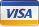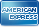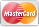•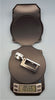•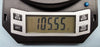# BETA DIGITAL PAPER BALANCE SCALE #100

by Beta Industries
Price \$ 180.00

# Beta Digital Paper Scale

This portable and accurate electronic scale makes it easy to determine the Grams per Square Meter (GSM) of any paper product.  If a square meter sample is available, simply crumple the sample into a ball and weigh it with the scale.

If only a smaller sample is available, measure it precisely and weigh it.  Multiply the displayed value in grams by the fraction of a square meter of the sample.

If your sample is one meter by one meter, multiply by 1.0

If your sample is 8.5 x 11 inches, multiply by 16.5775

If your sample is 10cm x 10cm, multiply by 100

• 100 gram capacity with 0.01 gram resolution
• Scale includes 50 and 100 Gram Calibration Weights
• Auto Zero
• Auto Power Off
• Low Battery Warning
• Convenient pocket size with soft case
• Overall dimensions 3.15 x 6 inches
(152 mm x 80 mm)
• Complete with 3v (2X LI) batteries